﻿ Forces and Newton's laws

# Dynamics: Forces and Newton's laws

Dynamics is about studying the forces and their effects on motion.

### Force

Force is a push or pull on an object. An object can exert a force on another object with a direct contact or without. If you push an object, you are applying a force on the object and there is a direct contact between you and the object. But when you drop an object, the earth pulls the object toward it, that is earth exert a force on the object without any direct contact. When a force is exerted on an object, the effect of the force on the object depends on both the size (magnitude) and the direction of the force. So force is a vector.

### Newton's Laws of Motion

There are three fundamental laws of motion formulated by Isaac Newton and was published in 1687. They are called, Newton's first law, Newton's second law and Newton's third law.

#### Newton's first law

It states that "when there is no net force acting on an object, an object in rest continues to stay in rest or an object in motion will continue its motion in a straight line."

An object can be in rest or in motion, that is its state. Changing the state of an object means, (i) if the object is in rest, you are making it to move; and (ii) if the object is in motion, you are changing its velocity. A velocity change can either be in magnitude or in direction. What Newton's first law says is, to change the state of an object, you must apply a net force on the object. Further, it says, if the state of the object is in motion, it will be in a straight line and to change that straight line path, you need to apply a force on the object.

All objects have a resistance to change its state. That resistance is called inertia of the object. Mass is the measure of inertia of an object. Heavier the object (more mass), stronger the resistance to change its state as more force is required for the change. Newton's first law is also known as the law of inertia.

#### Newton's second law

This law relates force and acceleration. Newton's second law states that "acceleration of an object is directly proportional to the net force acting on it, and inversely proportional to the object's mass."
$\vec{a}=\dfrac{\vec{F}}{m}$
where $\vec{F}$ is the net force on the object, net force means sum of all the forces acting on the subject. $m$ is the mass of the object.

The first law says that if you want to accelerate (i.e., to change the velocity) an object, you must apply a force. With the second law, you can calculate that acceleration. If an object is moving with a constant velocity, then there is no acceleration. No acceleration means, net force is zero. So, a force is not required to keep an object in motion. Force is only required to accelerate an object.

Newton's second law is generally written in this form:

$\vec{F}={m}\vec{a}$

The SI unit of mass is $kg$ and that of acceleration is $m/s^2$, so the unit of force will be $kgm/s^2$. We take, $kgm/s^2 = N$, where $N$ stands for newton. So, we use newton ($N$) as the unit of force. How much is $1N$ of force ? it is the force required to accelerate an object of mass, $1kg$ to $1m/s^2$.

#### Newton's third law

It states that "whenever an object exerts a force on a second object, the second object exerts an equal amount of force in the opposite direction on the first."

Newton's third law can also be stated as, "for every action there is equal and opposite reaction."

According to Newton's third law, force always acts in pair: force and its reaction force. The force and the reaction force are the same regardless of the size (mass) of the objects involved. For example if a small car and a big truck involve in a collision, whatever the force the truck exerts on the car is same as the force the car exerts on the truck, but the direction of the forces are opposite. Although the forces are the same, the smaller object is accelerated at a higher rate due to its smaller mass according to Newton's second law.

### Force of gravity (weight)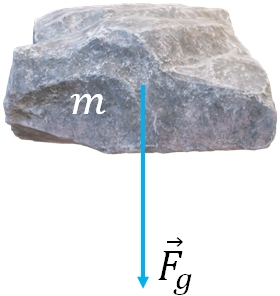Force of gravity on a falling object. Earth pulls objects toward it due to its gravity. This gravitational pull of the earth is called force of gravity. Any falling object near the surface of the earth, has an acceleration, $g$ as you learned in kinematics. Since gravity is the only force acting on a falling object (ignoring the air resistance), the net force on a falling object is just the force of gravity.Therefore, from Newton's second law, the force of gravity on a falling object of mass $m$ is
$F_g=mg$

When a falling object hits the ground, the earth doesn't stop puling the object. Earth still pulls the object with the same force but the Earth's surface keep it from moving further. So, the force of gravity is always acting on an object, whether the object is in motion or in rest. Since the Earth pulls the objects toward its center, the direction of the force of gravity is always vertically downward. Force of gravity is also called weight.

### Normal force

If you have an object on a horizontal surface, the surface exerts a force on the object, called normal force, $F_n$. Normal force always acts perpendicular to the surface. On a horizontal surface, the normal force balances the force of gravity and keeps the object from falling down.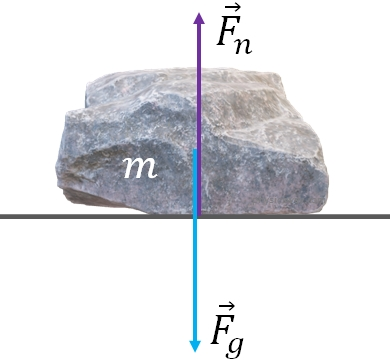Normal force on an object by a surface. So, on a horizontal surface, with no other forces acting on the object:
$F_n=mg$

If you put an object on a scale, what the scale reads is the normal force. That is the normal force exerted on the object by the surface of the scale. If the scale is in rest, then the normal force balances the weight of the object and it shows the actual weight of the object. You can realize this by standing in an elevator. If you are standing on a bathroom scale in an elevator, you will feel more weight and also the scale reads more weight, when the elevator is accelerating upwards. And you will feel less weight if it is accelerating downwards and also the scale reading will be lower. Normal force is also called apparent weight, while the actual weight is the force of gravity.

Scales normally do not show the readings in the unit of force. But it is calibrated to show the mass, in kg or lb, which is nothing but the normal force on the object divided by $g$. We call this apparent mass ($m'$) of the object, which can change but the actual mass $m$ does not. If the scale is in rest with the object, then a calibrated scale will show you the actual mass, $m$ of the object.

### Tension or force of tension:

If you use a flexible cord to pull an object, then you are applying a force to the object via the cord. Such a force is called force of tension or just tension, $F_t$. Tension is a pulling force transmitted through a flexible chord such as a cable, rope or a chain.

### Free body diagram

A diagram that shows all the forces acting on an object is called free body diagram or force diagram. When solving problems in dynamics, the first step is to draw the free body diagram. The free body diagram should show the directions (arrows) and the approximate magnitudes (length) of the forces. A free body diagram of an object on a surface is given above. The object is pulled to the right by an applied force $F_A$. From the length of the line that represents the force, $F_A$, you can tell that applied force is not as strong as the other two forces.

In the above, there is only one object. Sometimes, you may have a system that contains more than one object. In such cases, you need to draw the force diagram for each object separately.

### Solving problems

You already learned that the components of a vector is independent of each other, i.e., $x$ component of a vector does not affect its $y$ component and vice versa. Since force is a vector, the same is true for forces: a $x$ component of a force only affect the horizontal motion (motion along the $x$ axis) and the $y$ component, the vertical motion.

So when we solve problems in dynamics, we will work with $x$ and the $y$ components of the forces separately. If there are multiple forces acting on an object, you need to add the forces along the $x$ axis together and apply Newton's second law and add all the force along the $y$ axis and apply Newton's second law, i.e.,

$F_x=ma_x$ and

$F_y=ma_y$

where $F_x$ and $F_y$ are the net forces along the $x$ and the $y$ direction.

Example:You have a box of mass 11 kg and is pulled by a force, $\vec F_p = 32 N$ at an angle of 25 degrees above the horizontal,

(a) find the normal force on the box, and

(b) find the acceleration of the box.

First step is to draw the free body diagram:

Second, check if each force is on one of the axes, if a force is not along one of the axes, find its $x$ and $y$ components.

$F_n$ and $F_g$ are along the $y$ axis. Since, $\vec F_p$ is neither along the $x$ axis nor along the $y$ axis, you need to find its $x$ and $y$ components. From the figure,

$F_{px}=F_p \cos (25)= 32 \cos(25)=29N$

and

$F_{py}=F_p \sin (25)= 32 \sin(25)=13.52N$

(a) Finding the normal force:

Since the normal force is along the $y$ axis, add all the forces along $y$ and apply Newton's second law along $y$

Net force along $y$ is

$F_y=F_n-mg+F_{py}$

[I put a $-$ sign for the force of gravity as it is downward and $+$ sign for $F_{py}$ as it is upward.]

Applying Newton's second law along $y$:

$F_y=ma_y$

Since there is no motion of the box along $y$ direction, $a_y=0$

Therefore, $F_y=0$

Or $F_n-mg+F_{py}=0$

Solving for $F_n$

$F_n= mg-F_{py}$

Substituting the values,

$F_n=94N$

(b) Finding the acceleration

Add all the forces along the $x$ axis, to get the net force along $x$. There is only one force along $x$,

$F_x=F_{px}=29N$

Applying Newton's second law along $x$:

$F_x=ma_x$

Sbstitute the known values and solve for $a_x$

$a_x= 2.6 m/s^2$.

#### Two hanging masses over a pulley

Here, there are two masses, $m_1$ and $m_2$ hanging from a rope and the rope runs over a pulley. Assume, $m_2 > m_1$.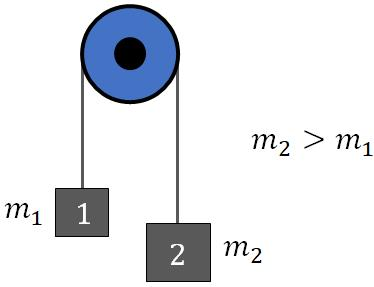Masses hanging from a rope over a pulley. When the masses are released, the mass $m_2$ will accelerate downward and $m_1$, upward. For simplicity, we assume that the pulley or the rope has negligible masses and the pulley exerts no friction on the rope. That makes the tension on both sides of the rope is the same. Since we have two objects here, you should draw two free body diagrams, one for each. Since $m_2$ is greater than $m_1$, the force of gravity on the object 2 is greater than that of 1. The length difference of the force vectors shows that.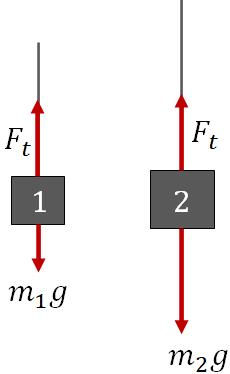Free body diagram - hanging masses.

### Friction:

When you try to slide an object on a surface, the microscopic bumps on the surface impede the motion. If the surface is smooth, we need less force to move the object, but a rough surface requires more force. Friction is a resistance that prevents an object in rest from moving when applying a force. If the object is in motion, then friction acts in the direction opposite to the direction of motion and try to stop the motion. Friction acts parallel to the surface.

#### Static friction:

Assume, you have an object on a horizontal surface in rest. You are trying to move the object by applying a horizontal force,$F_A$ . Your force is not strong enough that the object is not moving. Since the object is in rest, the net force on the object must be zero. Now you can realize that there must be another horizontal force of same magnitude as the applied force acting on the object in the opposite direction so that the net force is zero. This opposing force is the force of friction. Since the object is not moving, we call this friction static friction. Static friction prevents an object from moving when applying a force.Friction on an object in rest. Static friction balances the applied force.

Now, if you increase the applied force, friction also increases to prevent the object from moving until the object starts moving. So, you have
$F_{fr}=F_A$, if the object is not moving.
If you keep on increasing the force, and at one point, the object starts moving. The force you apply when the object just starts moving is the maximum friction.

Experimentally it has been found that the maximum friction is proportional to the normal force on the object by the surface:
i.e., ${(F_{fr})}_{max}=\mu_sF_n$,where $\mu_s$ is called the coefficient of static friction between the object and the surface. $\mu_s$ depends on the surface and the object.

#### Kinetic friction:

If the applied force on an object exceeds the maximum friction, then the object starts moving. Once the object start moving, friction reduces by some amount. Force of friction acting on an object in motion is called kinetic friction. Kinetic friction acts in the direction opposite to the direction of motion.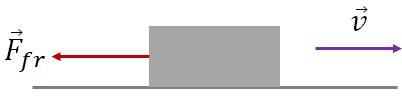Friction acts in the direction opposite to the direction of motion. Kinetic friction is proportional to the normal force exerted on the object by the surface.
$F_{fr}=\mu_kF_n$, where $\mu_k$ is the coefficient of kinetic friction between the object and the surface.

$\mu_s$ is always greater than $\mu_k$. Sometimes, we say some surface as smooth: a smooth surface means friction on the surface is zero and you should ignore the friction force on an object on that surface.

### Inclines:

An incline is a flat surface tilted relative to the horizontal. One end of the incline is higher than the other.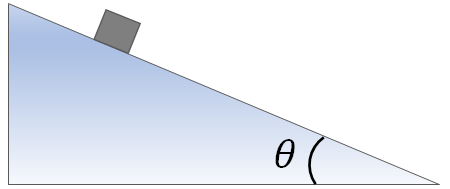An object on an incline plane Since the normal force on an object on a surface is perpendicular to the surface, on an incline, the normal force is not vertical. So, the normal force does not align with the force of gravity. Remember that force of gravity always acts vertically downward no matter on what surface the object is. On an incline, we take the $x$ axis is along the inclined surface and the $y$ axis is perpendicular to the surface so that we have the normal force aligned with the $y$ axis as usual.
An inclined surface can be smooth with no friction or there may be friction. If there is no friction, then the object cannot be in rest on an incline as the force of gravity will bring it down.
Force diagram for an object on a smooth (no friction) inclined surface is given the following figure.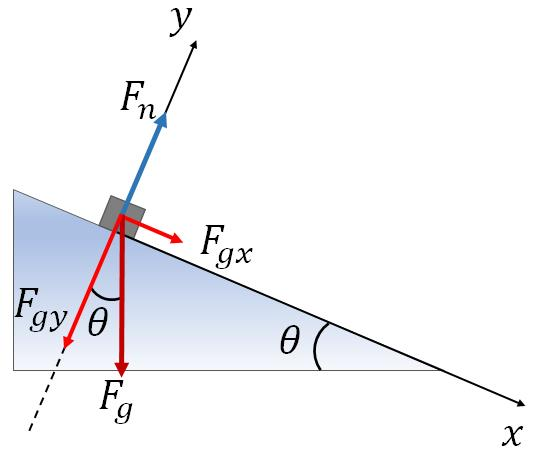Forces acting on an object on an incline plane. Force of gravity of an object on an incline is neither along the $y$ axis nor along the $x$ axis. Therefore, we need to find its $x$ and $y$ components. From the geometry of the figure, we have
$F_{gx}=mg\sin\theta$
$F_{gy}=mg\cos\theta$
On an incline, motion of the object if any can take place only along the $x$ axis, and there is no motion along the $y$ axis. Along the $y$ direction, normal force balances the $y$ component of the force of gravity,
$F_n=F_{gy}=mg\cos\theta$.
Objects in rest on an incline: For an object to be stay rest on an incline, the maximum friction on the object by the surface should be greater than the $x$ component of the force of gravity. When the object is in rest, force of friction balances $F_{gx}$:
$F_{fr}=F_{gx}=mg\sin\theta$.
Object's motion on an incline:On an incline, an object can be either sliding down or going up with some given initial velocity. This motion is one dimensional, so we can use the 1-D kinematic equations to study it. We will take the positive direction ($+x$) towards the downhill. So if the object is sliding up, it is moving in the $-x$ direction and its initial velocity will be negative (i.e., you need to add a minus sign to the initial speed). If the surface is smooth, you ignore the friction. But if there is friction, then its acts in the direction opposite to the direction of the object's motion. If the object is sliding down, then the friction acts in the up hill direction and vice versa. To study the kinematics of an object on an incline, you need to find the object's acceleration, which can be obtained by applying Newton's second law.

Following is the free body diagram of an object on an incline that is sliding down on the incline. The surface is not smooth and has friction. Since the object is sliding down, the friction acts in the uphill direction to oppose the motion.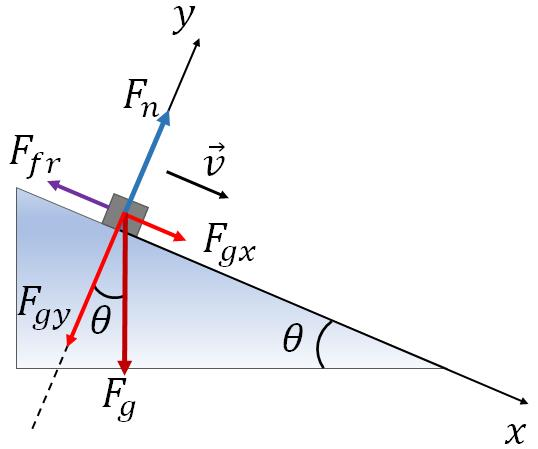Free body diagram of an object on incline that is sliding down. In the above figure, applying Newton's second law along the $x$ axis, we have
$-F_{fr}+mg\sin\theta=ma$
With the normal force and the coefficient of kinetic friction, you can find the friction,
$F_{fr}=\mu_k\:F_n=\mu_k\:mg\cos\theta$.
Substituting this in the above equation and dividing out the mass, $m$, you will get,
$a=-\mu_k\:g\cos\theta+g\sin\theta$
This is the acceleration of the object that is sliding down.
Once you know the acceleration, you can study the motion of the object, such as how long an object will take to slide down from the top of the incline. You will use the 1-D kinematic equation to study the motion.

Note that the direction of the friction force is opposite to the direction of motion. So, if an object is moving upward (uphill), then the friction will be downward (downhill)